Open in App
Not now

# Class 9 NCERT Solutions – Chapter 6 Lines And Angles – Exercise 6.2

• Difficulty Level : Hard
• Last Updated : 18 Jan, 2022

### Question 1. In given figure, find the values of x and y and then show that AB || CD.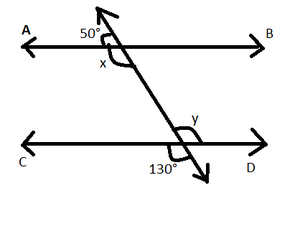Solution:

After given names to the remaining vertices we get,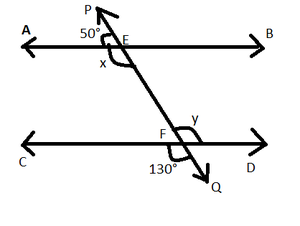Now, Given ∠AEP = 50°, ∠CFQ = 130°

=> ∠EFD = ∠CFQ  [vertically opposite angles are equal]

=> y = 130°   [Given ∠CFQ = 130°]

=> y = 130°  —eq(i)

Now, PQ is taking as straight line so, sum of all angles made on it is 180°

=> ∠AEP  + ∠AEQ = 180°

=> 50° + x = 180°

=> x = 180° – 50° = 130°

=> x = 130° –eq(ii)

Now, from eq(i) and eq(ii) We conclude that x = y

As they are pair of alternate interior angles

So, AB || CD

Hence proved!!!

### Question 2. In given figure, if AB || CD, CD || EF and y : z = 3 : 7, find x.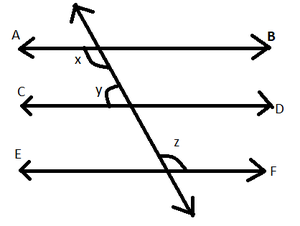Solution:

Given AB || CD and CD || EF  y : z = 3 : 7

=> AB || CD || EF

=> AB || EF

So, x=z [alternate interior angles] –eq(i)

Again AB || CD

=> x + y = 180° [Co-interior angles]

=> z + y = 180°  –eq(ii) [from eq(i)]

But given that y : z = 3 : 7

=> z = (7/3) y = (7/3)(180° – z) [from eq(ii)]

=> 10z = 7 * 180°

=> z = (7 * 180°)/10 =126°

=> z  = 126° –eq(iii)

from eq(i) and eq(iii) we have

=> x = 126°

### Question 3. In given figure, if AB || CD, EF ⊥ CD and ∠GED = 126°, find ∠AGE, ∠GEF and ∠FGE.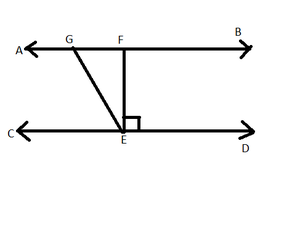Solution:

Given AB || CD, EF ⊥ CD, ∠GED = 126° and ∠FED =90°

=> ∠GED =  ∠GEF + ∠FED

=> 126° = ∠GEF + 90°  [Given]

=> ∠GEF = 36°

As, AB || CD and GE is a transversal

So, ∠FGE + ∠GED = 180° [ sum of Co- interior angles is 180 ]

=>  ∠FGE + 126° = 180° [ Given ]

=>  ∠FGE = 54°

As, AB || CD and GE is a transversal

So, ∠AGE = ∠GED [ alternate angles are equal ]

=> ∠AGE = 126°

### Question 4. In given figure, if PQ || ST, ∠PQR = 110° and ∠RST = 130°, find ∠QRS.

[Hint: Draw a line parallel to ST through point R.]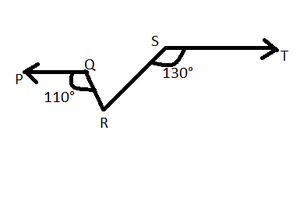Solution:

Firstly we have drawn a line EF parallel to ST (EF || ST)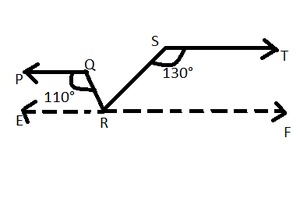Since, PQ || ST [Given] and EF || ST [ Construction ]

So, PQ || EF and QR is a transversal

=> ∠PQR = ∠QRF [ Alternate interior angles ]

=> ∠QRF = 110°   [Given ∠PQR =110° ]

=> ∠QRF = ∠QRS + ∠SRF

=> ∠QRS + ∠SRF = 110°  –eq(i)

Again ST || EF and RS is a transversal

=>∠RST + ∠SRF = 180° [ sum of  Co-interior angles is 180° ]

=>130° + ∠SRF =180° [Given]

=>∠SRF =50°

Now , from eq(i)

=> ∠QRS + ∠SRF = 110°

=> ∠QRS +50 = 110°

=> Thus, ∠QRS = 60°

### Question 5. In Fig. 6.32, if AB || CD, ∠ APQ = 50° and ∠ PRD = 127°, find x and y.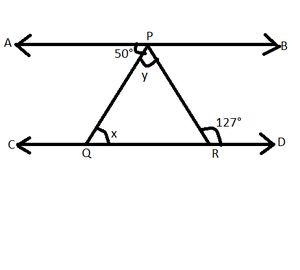Solution:

Given  AB || CD and PQ is a transversal

=> ∠APQ = ∠PQR [ Alternate interior angles ]

=> x= 50° [ Given ∠APQ = 50° ]

=> x = 50°

Again, AB || CD and PR is a transversal

=>∠APR = ∠PRD [ Alternate interior angles ]

=> ∠APR = 127° [ Given ∠PRD = 127° ]

=> 50° + y =127° [Given ∠APQ = 50° ]

=> y =127° – 50° = 77°

=>  y = 77°

Thus,  x = 50° and y = 77°

### Question 6. In given figure, PQ and RS are two mirrors placed parallel to each other. An incident ray AB strikes the mirror PQ at B, the reflected ray moves along the path BC and strikes the mirror RS at C and again reflects back along CD. Prove that AB || CD.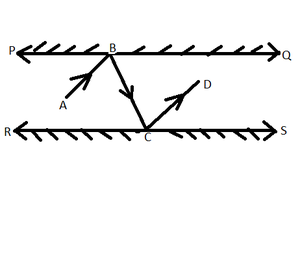Solution:

Draw ray BL ⊥ PQ and CM ⊥ RS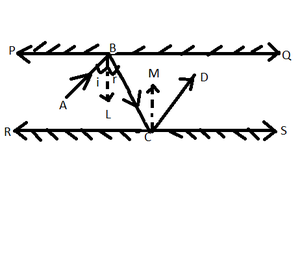Since, PQ || RS => BL || CM

=>[ So, BL || PQ and CM || RS ]

Now, BL || CM and BC is a transversal

=> ∠LBC = ∠MCB –eq(i) [ Alternate interior angles ]

Since, angle of incidence = angle of reflection

=>  ∠ABL = ∠LBC  and  ∠MCB = ∠MCD

=> ∠ABL = ∠MCD –eq(ii) [By eq(i)]

=>Adding eq(i) and eq(ii) we get

=>  ∠LBC + ∠ABL = ∠MCB + ∠MCD

=> ∠ABC = ∠BCD

i.e, a pair of alternate angles are equal

Thus, AB || CD

Hence, Proved !!!

My Personal Notes arrow_drop_up